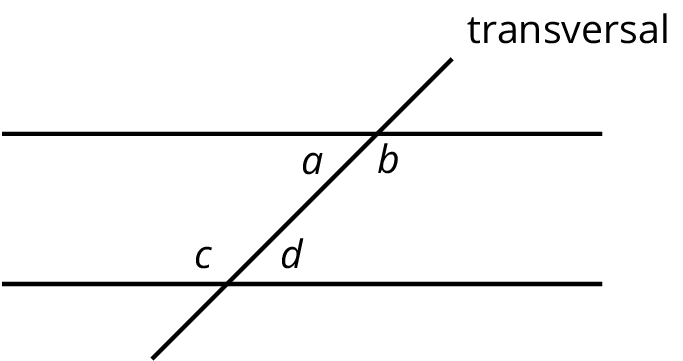# Lesson 12

Alternate Interior Angles

Let’s explore why some angles are always equal.

### 12.1: Angle Pairs

1. Find the measure of angle $$JGH$$. Explain or show your reasoning.2. Find and label a second $$30^\circ$$ angle in the diagram. Find and label an angle congruent to angle $$JGH$$.
3. Angle $$BAC$$ is a right angle. Find the measure of angle $$CAD$$.​​​​​

### 12.2: Cutting Parallel Lines with a Transversal

Lines $$AC$$ and $$DF$$ are parallel. They are cut by transversal $$HJ$$.

1. With your partner, find the seven unknown angle measures in the diagram. Explain your reasoning.

2. What do you notice about the angles with vertex $$B$$ and the angles with vertex $$E$$?
3. Using what you noticed, find the measures of the four angles at point $$B$$ in the second diagram. Lines $$AC$$ and $$DF$$ are parallel.

4. The next diagram resembles the first one, but the lines form slightly different angles. Work with your partner to find the six unknown angles with vertices at points $$B$$ and $$E$$.

5. What do you notice about the angles in this diagram as compared to the earlier diagram? How are the two diagrams different? How are they the same?

Parallel lines $$\ell$$ and $$m$$ are cut by two transversals which intersect $$\ell$$ in the same point. Two angles are marked in the figure. Find the measure $$x$$ of the third angle.

### 12.3: Alternate Interior Angles Are Congruent

Lines $$\ell$$ and $$k$$ are parallel and $$t$$ is a transversal. Point $$M$$ is the midpoint of segment $$PQ$$.

Find a rigid transformation showing that angles $$MPA$$ and $$MQB$$ are congruent.

2. In this picture, lines $$\ell$$ and $$k$$ are no longer parallel. $$M$$ is still the midpoint of segment $$PQ$$.

Does your argument in the earlier problem apply in this situation? Explain.

### 12.4: Info Gap: Angle Finding

Your teacher will give you either a problem card or a data card. Do not show or read your card to your partner.

If your teacher gives you the problem card:

3. Explain how you are using the information to solve the problem.

Continue to ask questions until you have enough information to solve the problem.

4. Share the problem card and solve the problem independently.

If your teacher gives you the data card:

2. Ask your partner “What specific information do you need?” and wait for them to ask for information.

If your partner asks for information that is not on the card, do not do the calculations for them. Tell them you don’t have that information.

3. Before sharing the information, ask “Why do you need that information?” Listen to your partner’s reasoning and ask clarifying questions.

4. Read the problem card and solve the problem independently.

5. Share the data card and discuss your reasoning.

### Summary

If two angle measures add up to $$90^\circ$$, then we say the angles are complementary. If two angle measures add up to $$180^\circ$$, then we say the angles are supplementary. When two lines intersect, vertical angles are equal and adjacent angles are supplementary. For example, in this figure angles 1 and 3 are equal, angles 2 and 4 are equal, angles 1 and 4 are supplementary, and angles 2 and 3 are supplementary.

When two parallel lines are cut by another line, called a transversal, two pairs of alternate interior angles are created. (“Interior” means on the inside, or between, the two parallel lines.) For example, in this figure angles 3 and 5 are alternate interior angles and angles 4 and 6 are also alternate interior angles.

Alternate interior angles are equal because a $$180^\circ$$ rotation around the midpoint of the segment that joins their vertices takes each angle to the other. Imagine a point $$M$$ halfway between the two intersections—can you see how rotating $$180^\circ$$ about $$M$$ takes angle 3 to angle 5?

Using what we know about vertical angles, adjacent angles, and alternate interior angles, we can find the measures of any of the eight angles created by a transversal if we know just one of them. For example, starting with the fact that angle 1 is $$70^\circ$$ we use vertical angles to see that angle 3 is $$70^\circ$$, then we use alternate interior angles to see that angle 5 is $$70^\circ$$, then we use the fact that angle 5 is supplementary to angle 8 to see that angle 8 is $$110^\circ$$ since $$180 -70 = 110$$. It turns out that there are only two different measures. In this example, angles 1, 3, 5, and 7 measure $$70^\circ$$, and angles 2, 4, 6, and 8 measure $$110^\circ$$.

### Glossary Entries

• alternate interior angles

Interior angles are angles that are made by a transversal crossing two parallel lines. They are the angles that lie between the parallel lines, not outside them.

If two interior angles lie on opposite sides of the transversal they are called alternate interior angles.

In the figure, $$a$$ and $$d$$ are alternate interior angles, and $$b$$ and $$c$$ are also alternate interior angles.• complementary

Complementary angles have measures that add up to 90 degrees.

For example, a $$15^\circ$$ angle and a $$75^\circ$$ angle are complementary.

• supplementary

Supplementary angles have measures that add up to 180 degrees.

For example, a $$15^\circ$$ angle and a $$165^\circ$$ angle are supplementary.

This diagram shows a transversal line $$k$$ intersecting parallel lines $$m$$ and $$\ell$$.